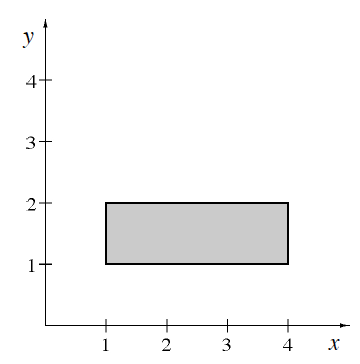### Home > APCALC > Chapter 2 > Lesson 2.3.2 > Problem2-121

2-121.

You have found (or approximated) the volume of a rotated flag with various shapes. What if the axis of rotation (the “pole”) is not attached directly to the flag?

In the graph at right, there is a gap between the flag and the x-axis. Decide what will occur when this flag is rotated about the $x$-axis. Draw a sketch of the result and calculate the volume of the resulting solid.

A large cylinder, with a hollow cylindrical hole in the center.

Compute two volumes and subtract: a large solid cylinder $−$ the cylindrical hole.

Both cylinders have a height of $3$.

The large cylinder has a radius of $2$. The small cylinder has a radius of $1$.Use the eTool below to visualize the flag.
Click the link at right for the full version of the eTool: Calc 2-121 HW eTool